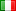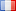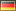X

# 36° Stormo Virtuale36STV0 / 500 user

38
15
0%

### User activity graph### User list

•=36=Drigo
•=36=Wolf(R)
•Lupus
•=36=Caronte(R)
•=36=Bountyhunter
•=36=Johnny
•=36=Hulioz(R)
•=36=Falcon
•=36=Airwolf(R)
•=36=Raider(R)
•=36=Ghost(R)
•=36=Psycho(R)
•=36=Arawindor
•=36=Heater(R)
•=36=Buch(R)
•=36=Artax(R)
•=36=Dirty
•=36=Scorpion
•=36=Tournament
•=36=Mav(R)
•=36=Rafiki(R)
•=36=Raf
•=36=Majo
•=36=Firo
•=36=Witcher(R)
•=36=Sniper(R)
•Crudo
•=36=Breath
•=36=Edokg